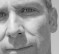## Whitening of a spatially correlated MIMO signalStarted by 4 months ago1 replylatest reply 3 months ago4 views

If I have a MIMO signal

$$y = H_{corr}x + w$$ where $w$ is a White Gaussian noise, and $H_{corr}$ is the effective MIMO channel matrix that consists of correlated channel coefficients.

Particularly, $$H_{corr} = L_{rx}H$$ where $L_{rx}$ is obtained as a Cholesky decomposition of the channel correlation matrix $R$, and $H$ is the MIMO effective matrix for an uncorrelated channel.

**Method 1**:

I can do a simple whitening transformation here by multiplying $y$ with the inverse of $L_{rx}$ to cancel the correlation in the channel. But by doing so, I am colouring the white noise also as $$L_{rx}^{-1}y = Hx + L_{rx}^{-1}w$$

Ideally, signal detectors assume the noise to be white and hence, this may result in bad error performance especially when the correlation coefficient $\rho$ is close to unity.

**Method 2**:

Another method is to whiten the effective signal by firstly obtaining the autocorrelation function of $y$.  The autocorrelation function then becomes equal to $$R + \sigma^2I$$. We then do a Cholesky decomposition of the autocorrelation function and multiply the signal with the inverse of the Cholesky decomposed matrix i.e. let's say $$\hat{L}_{rx}^{-1}$$, where $$\hat{L}_{rx}\hat{L}_{rx}^\dagger = R + \sigma^2I$$.

That way, the effective signal ($$\hat{L}_{rx}^{-1}y$$) becomes white. Although the effective signal becomes white, the individual signal and noise terms do not get whitened separately. In that case, during signal detection, the noise is still coloured and this may not necessarily give good error performance.

**My question**

Is there a way to estimate the signal and the noise term separately from the received signal which is effectively white? Or any other method to take care of the colouring of the noise?

Thanks

[ - ]